# I Intro to Statistics II Various Variables III

• Slides: 33
Download presentation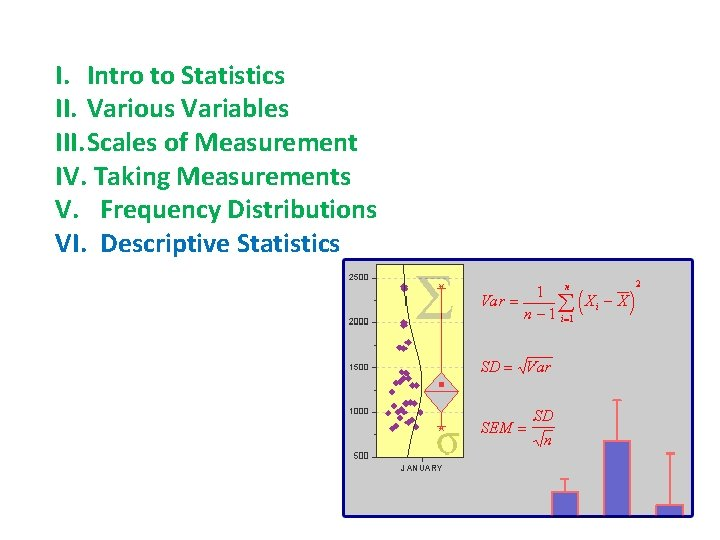I. Intro to Statistics II. Various Variables III. Scales of Measurement IV. Taking Measurements V. Frequency Distributions VI. Descriptive Statistics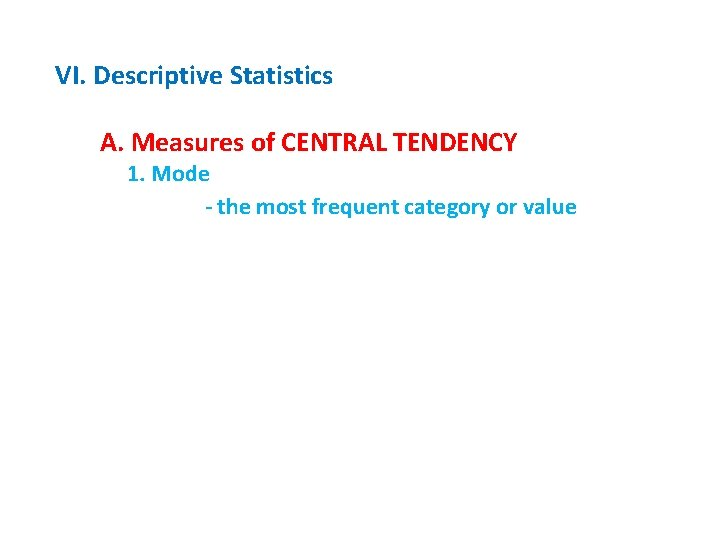VI. Descriptive Statistics A. Measures of CENTRAL TENDENCY 1. Mode - the most frequent category or valueVI. Descriptive Statistics A. Measures of CENTRAL TENDENCY 1. Mode 2. Median - the middle value in ordered, ranked data - represented by q for population - represented by M for a sampleVI. Descriptive Statistics A. Measures of CENTRAL TENDENCY 1. Mode 2. Median 3. Mean - arithmetic average; most common measure of central tendency for interval/ratio scale.VI. Descriptive Statistics A. Measures of CENTRAL TENDENCY 1. Mode 2. Median 3. Mean 4. Comparisons - the mean is the most ‘information rich’, as it is affected by every value in the population/sample. - but because of this, it is more affected by extreme values.Examples: Consider a sample were the mode is ‘ 10’ (or ‘red’) because there are 15 cases of this value (or attribute). This descriptor of central tendency doesn’t change if there are 5 ‘ 9’s’, 8 ‘ 9’s’, 6 ‘ 1020’s’, or whatever; as long as no value has a greater frequency. 15 Doesn’t matter; as long as frequencies are less than 15. 10 RED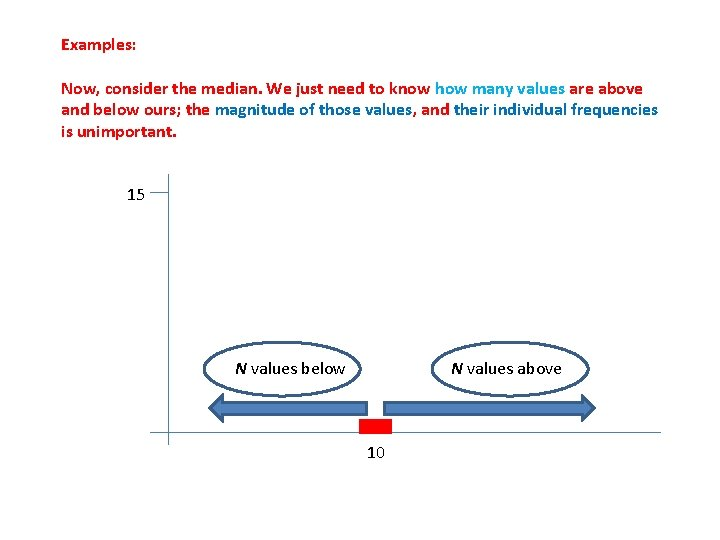Examples: Now, consider the median. We just need to know how many values are above and below ours; the magnitude of those values, and their individual frequencies is unimportant. 15 N values below N values above 10Examples: Now, consider the mean. The value of each case is included in the computation, so the mean is affected by each value. Changes in the magnitude and frequency of values may change the mean.Ranked Salaries in 1000’s: 15 17 21 21 24 27 27 31 225 Mode = 21, 27 (bimodal) Median = 24 Mean = 45. 33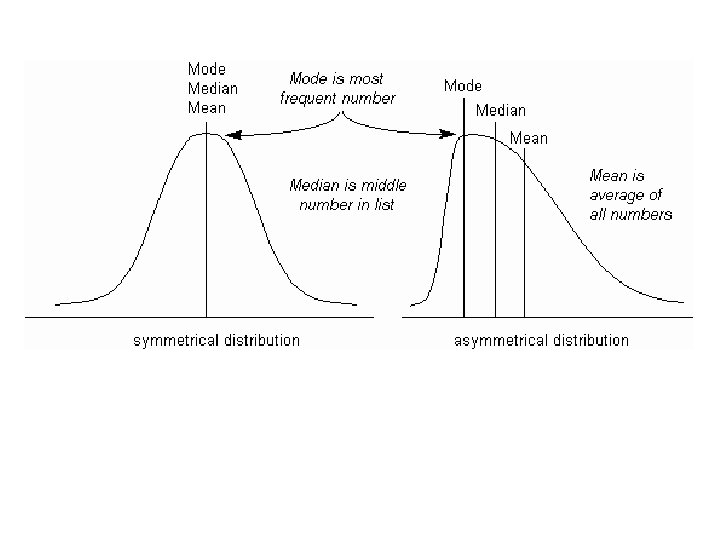VI. Descriptive Statistics A. Measures of CENTRAL TENDENCY 1. Mode 2. Median 3. Mean 4. Comparisons 5. Weighted mean – when different items have different degrees of importance (weight).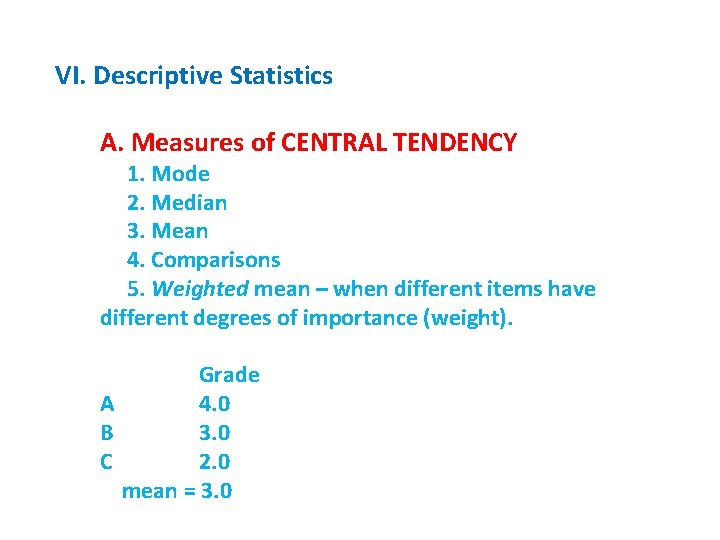VI. Descriptive Statistics A. Measures of CENTRAL TENDENCY 1. Mode 2. Median 3. Mean 4. Comparisons 5. Weighted mean – when different items have different degrees of importance (weight). Grade A 4. 0 B 3. 0 C 2. 0 mean = 3. 0VI. Descriptive Statistics A. Measures of CENTRAL TENDENCY 1. Mode 2. Median 3. Mean 4. Comparisons 5. Weighted mean – when different items have different degrees of importance (weight). A B C Grade 4. 0 3. 0 2. 0 GPA = 22/8 = 2. 75 Credits 2. 0 (May. X) 4. 0 Sum = 8. 0 grade pts 8. 0 6. 0 8. 0 22. 0VI. Descriptive Statistics A. Measures of CENTRAL TENDENCY B. Location in a SAMPLE/POPULATION - median – the midpoint in a distribution also the “ 50 th percentile” ½ of the values above and belowVI. Descriptive Statistics A. Measures of CENTRAL TENDENCY B. Location in a SAMPLE/POPULATION - median – the midpoint in a distribution also the “ 50 th percentile” ½ of the values above and below - 1 st quartile = 25 th percentile: 25% below. - 2 nd quartile = 50 th percentile - 3 rd quartile = 75 th percentile - 90 th percentile = 90% below.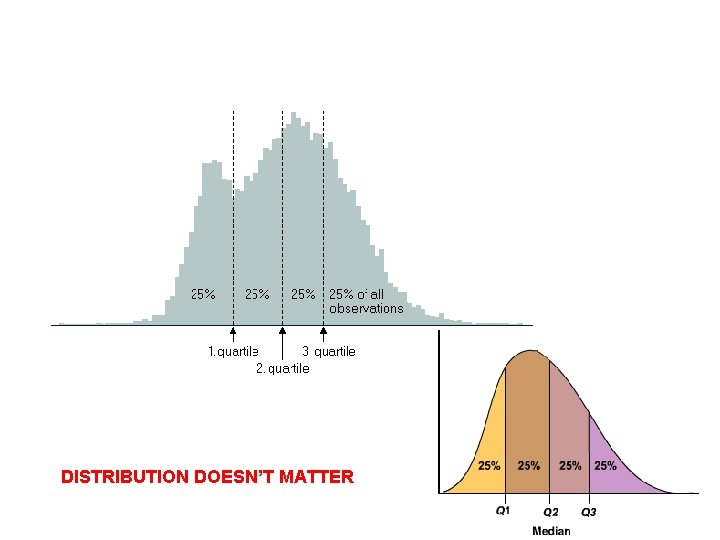DISTRIBUTION DOESN’T MATTER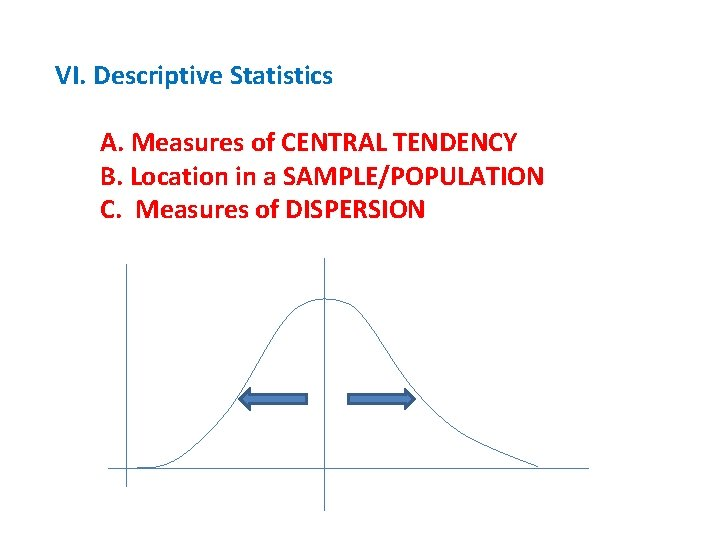VI. Descriptive Statistics A. Measures of CENTRAL TENDENCY B. Location in a SAMPLE/POPULATION C. Measures of DISPERSIONVI. Descriptive Statistics A. Measures of CENTRAL TENDENCY B. Location in a SAMPLE/POPULATION C. Measures of DISPERSION 1. Range: affected by only two values, and the most EXTREME values. - statistically – may be of little use - biologically – may be important as physiological/ ecological tolerance limits.VI. Descriptive Statistics A. Measures of CENTRAL TENDENCY B. Location in a SAMPLE/POPULATION C. Measures of DISPERSION 1. Range: affected by only two values, and the most EXTREME values. 2. Interquartile Range: from 1 st to 3 rd quartile.3. Standard Deviation (s, s): The average distance between data points and the mean. xi - x DATA: 1, 2, 2, 3, 3, 4, 4, 5 MEAN: 24/8 = 3 1 – 3 = -2 2 – 3 = -1 2 – 2 = -1 3– 3=0 4– 3=1 5– 3=2 S = (0) 4 1 1 0 0 1 1 4 S = (xi – x)2 = 12 THIS IS ALSO CALLED THE “SUM OF SQUARES”3. Standard Deviation (s, s): The average distance between data points and the mean. xi - x DATA: 1, 2, 2, 3, 3, 4, 4, 5 MEAN: 24/8 = 3 1 – 3 = -2 2 – 3 = -1 2 – 2 = -1 3– 3=0 4– 3=1 5– 3=2 S = (0) 4 1 1 0 0 1 1 4 Variance = S 2 = (ss)/n-1 ss = (xi – x)2 = 12 THIS IS ALSO CALLED THE “SUM OF SQUARES”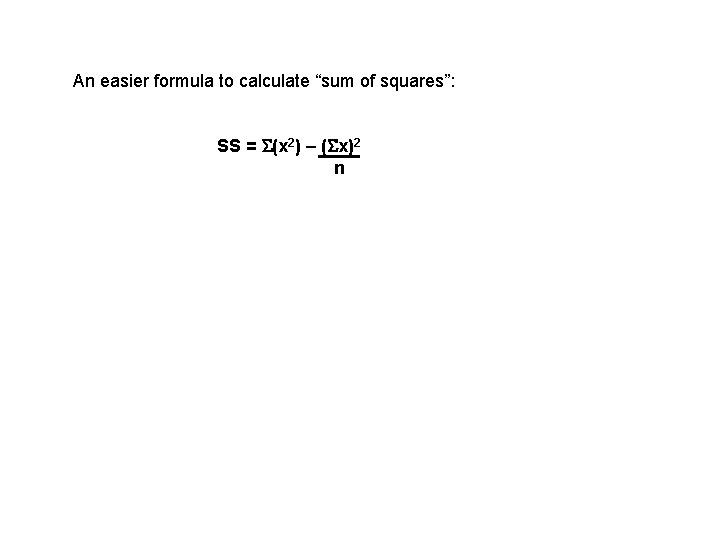An easier formula to calculate “sum of squares”: SS = S(x 2) – (Sx)2 n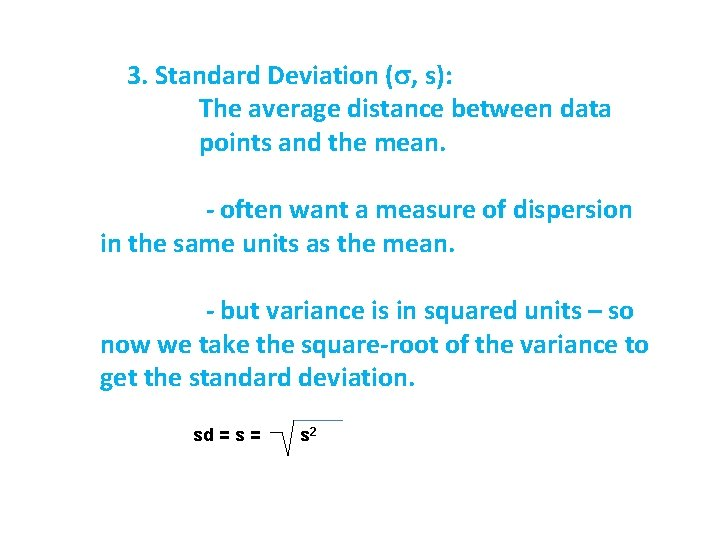3. Standard Deviation (s, s): The average distance between data points and the mean. - often want a measure of dispersion in the same units as the mean. - but variance is in squared units – so now we take the square-root of the variance to get the standard deviation. sd = s 2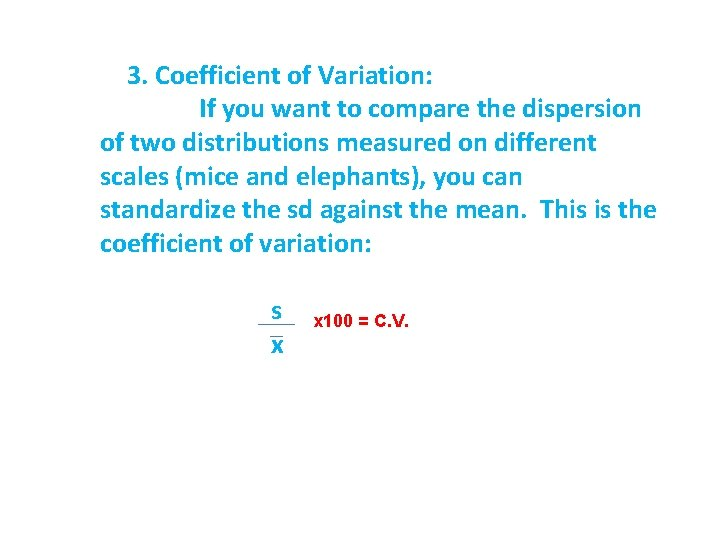3. Coefficient of Variation: If you want to compare the dispersion of two distributions measured on different scales (mice and elephants), you can standardize the sd against the mean. This is the coefficient of variation: s x x 100 = C. V.WRITING PAPERS I. General Comments II. Structure and Scope of a Review Paper III. Structure of a Primary Research Paper A. Abstract B. Introduction C. MethodsC. Methods - What you did and why, in enough detail so it can be replicated - Written in prose – paragraphs - logical flow… maybe temporal, maybe not. Maybe presented in association with specific questions - Past tense… active / passive voice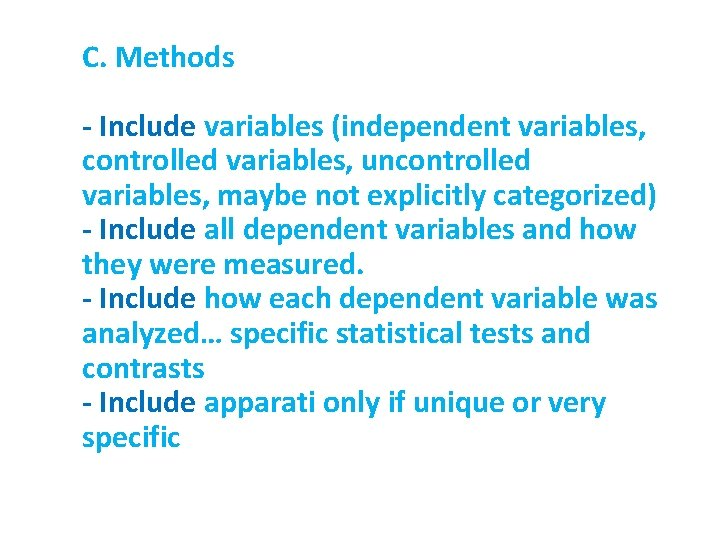C. Methods - Include variables (independent variables, controlled variables, uncontrolled variables, maybe not explicitly categorized) - Include all dependent variables and how they were measured. - Include how each dependent variable was analyzed… specific statistical tests and contrasts - Include apparati only if unique or very specific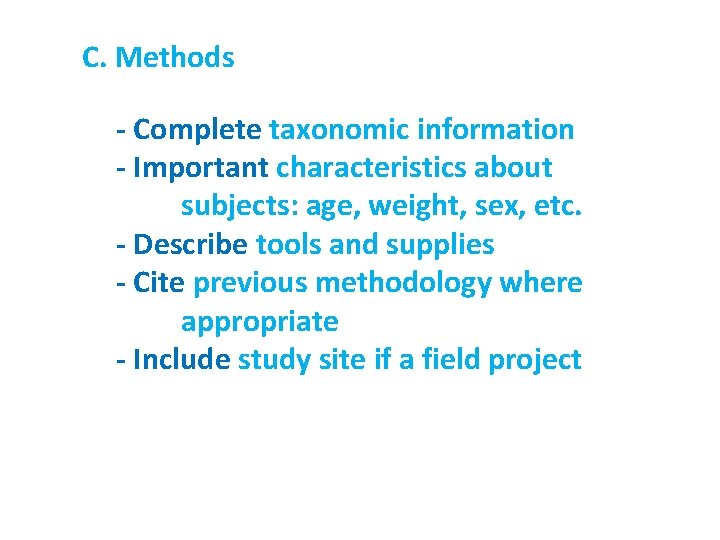C. Methods - Complete taxonomic information - Important characteristics about subjects: age, weight, sex, etc. - Describe tools and supplies - Cite previous methodology where appropriate - Include study site if a field projectWRITING PAPERS I. General Comments II. Structure and Scope of a Review Paper III. Structure of a Primary Research Paper A. Abstract B. Introduction C. Methods D. Results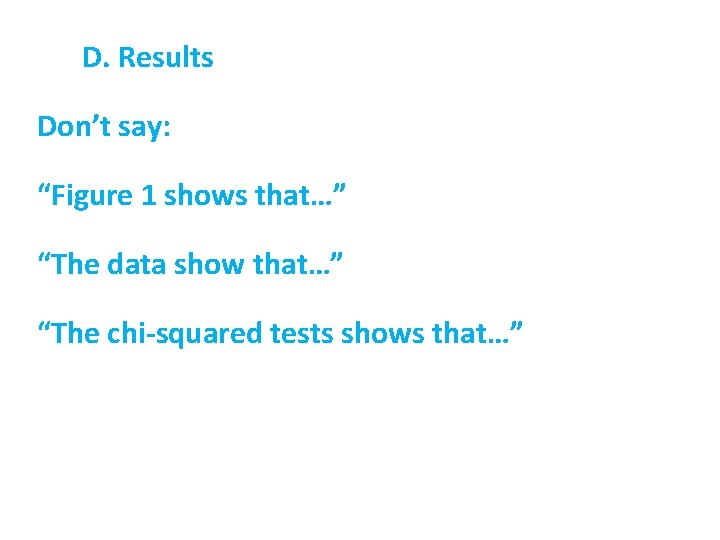D. Results Don’t say: “Figure 1 shows that…” “The data show that…” “The chi-squared tests shows that…”D. Results Don’t say: “Figure 1 shows that… mass is inversely correlated with density” “The data show that… abundance declines with density” “The chi-squared tests shows that… the gene exhibits complete dominance. ”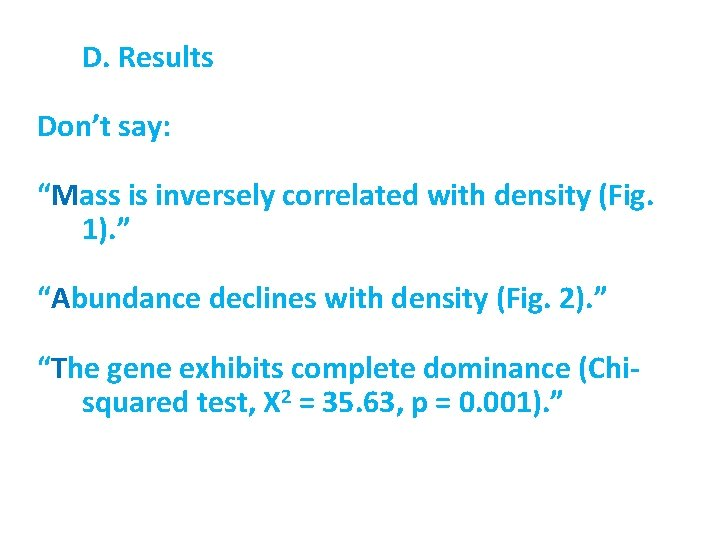D. Results Don’t say: “Mass is inversely correlated with density (Fig. 1). ” “Abundance declines with density (Fig. 2). ” “The gene exhibits complete dominance (Chisquared test, X 2 = 35. 63, p = 0. 001). ”